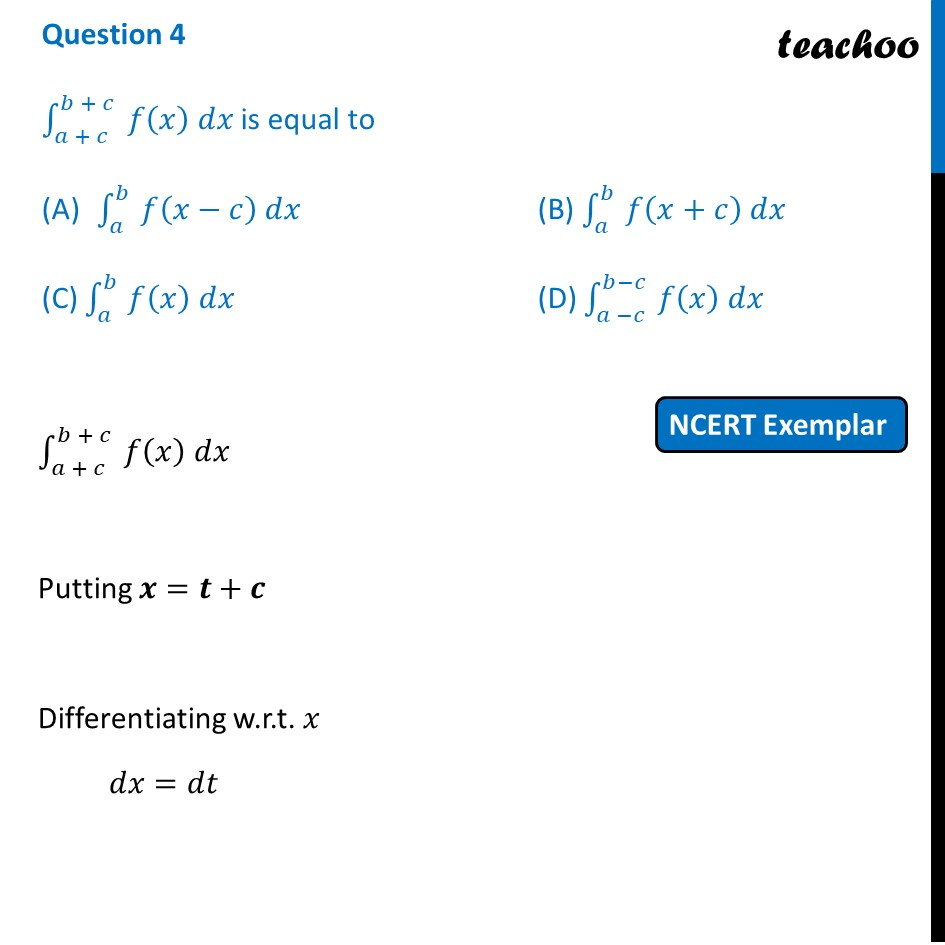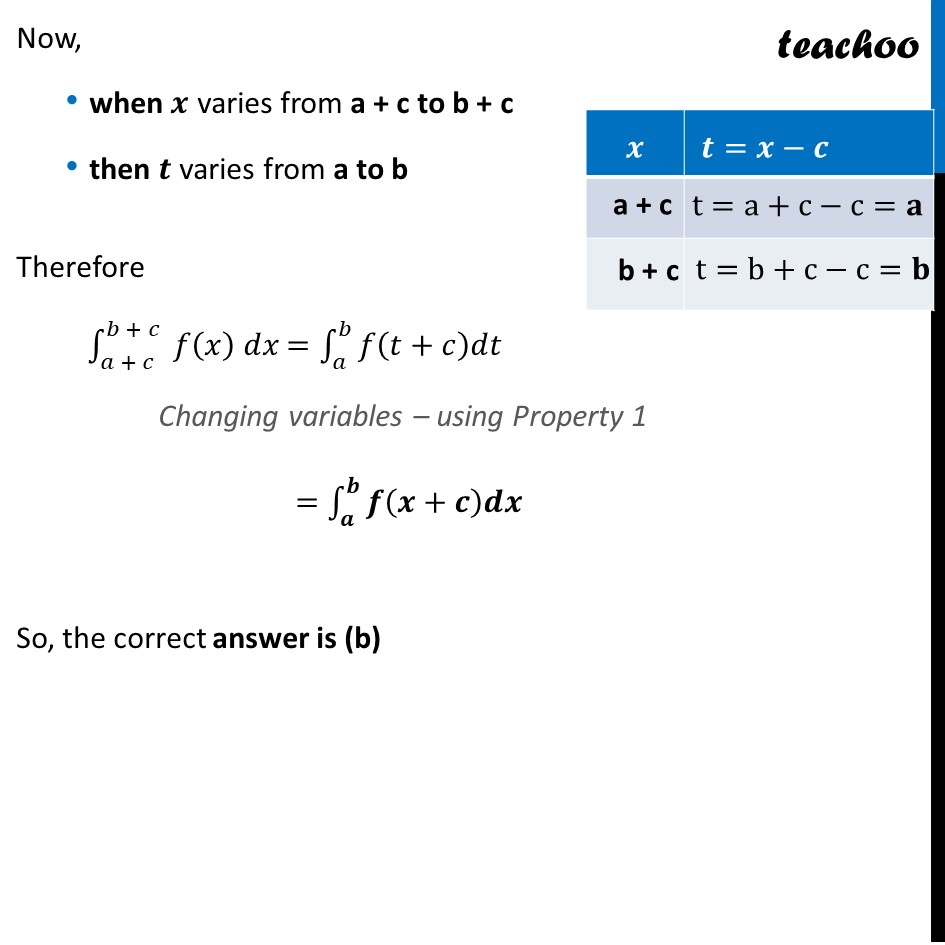NCERT Exemplar MCQ

Chapter 7 Class 12 Integrals
Serial order wise

## (D) ∫ (a - c)^(b - c) (x) dx

This question is similar to Misc 43 (MCQ) - Chapter 7 Class 12 - IntegralsLearn in your speed, with individual attention - Teachoo Maths 1-on-1 Class

### Transcript

Question 4 ∫1_(𝑎 + 𝑐)^(𝑏 + 𝑐)▒〖 𝑓(𝑥) 𝑑𝑥〗 is equal to ∫1_(𝑎 )^(𝑏 )▒〖𝑓(𝑥−𝑐) 𝑑𝑥〗 (B) ∫1_(𝑎 )^(𝑏 )▒〖𝑓(𝑥+𝑐) 𝑑𝑥〗 (C) ∫1_(𝑎 )^(𝑏 )▒〖𝑓(𝑥) 𝑑𝑥〗 (D) ∫1_(𝑎 −𝑐)^(𝑏−𝑐 )▒〖𝑓(𝑥) 𝑑𝑥〗 ∫1_(𝑎 + 𝑐)^(𝑏 + 𝑐)▒〖 𝑓(𝑥) 𝑑𝑥〗 Putting 𝒙=𝒕+𝒄 Differentiating w.r.t. 𝑥 𝑑𝑥=𝑑𝑡 Now, when 𝒙 varies from a + c to b + c then 𝒕 varies from a to b Therefore ∫1_(𝑎 + 𝑐)^(𝑏 + 𝑐)▒〖 𝑓(𝑥) 𝑑𝑥〗 =∫_𝑎^𝑏▒𝑓(𝑡+𝑐)𝑑𝑡 Changing variables – using Property 1 =∫_𝒂^𝒃▒𝒇(𝒙+𝒄)𝒅𝒙 So, the correct answer is (b)